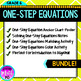# Solving One-Step Equations Notes, Poster, Matching, and Coloring BUNLDE!Subject
Resource Type
File Type

Zip

(6 MB|8 pages)
Product Rating
4.0
(2 Ratings)
Standards
4 Products in this Bundle
4 products
1. Identifying Inverse Operations of One-Step Equations Matching ActivityThis matching activity is a great way to practice solving one-step equations using inverse operations. Students will match each one-step equation to the correct step to solve the equation. For example "x+2=5 in order to solve th
2. One-Step Equations Color by Number Math Activity (Perfect for St. Patrick's)This color by number activity is a fun and engaging way for students to practice solving one-step equations using inverse operations. Students will evaluate 10 one-step equations and using inverse operations (addition, subt
3. Solving One-Step Equations Anchor Chart PosterThis anchor chart poster is a great tool to display in your classroom or hand out to students to put in their notebooks or binder. This anchor chart displays how to solve one-step equations using inverse operations. It also includes important vocabular
4. Solving One-Step Equations Interactive Notes (All Four Operations)This foldable is guided notes for the solving one-step equations. Students will identify the inverse operation for each one-step equation and properly isolate the variable. These interactive notes include a one-step equation example
• Bundle Description
• StandardsNEW

1) One-Step Equations Color by Number Math Activity

This color by number activity is a fun and engaging way for students to practice solving one-step equations using inverse operations. Students will evaluate 10 one-step equations and using inverse operations (addition, subtraction, multiplication, and division. Each incorrect answer is thoughtfully considered if students do not correctly use the inverse operation. This activity is aligned with the sixth (6th) grade common core standards. . This color by number activity is perfect for St. Patrick's Day or anytime during the year!

WHATS INCLUDED:

★ 10 one-step equations using addition, subtraction, multiplication and division.

★ Fractions and Decimals are included.

*********************************************************************************************************

2) Solving One-Step Equations Interactive Notes (All Four Operations)

This foldable is guided notes for the solving one-step equations. Students will identify the inverse operation for each one-step equation and properly isolate the variable.
These interactive notes include a one-step equation example for addition, subtraction, multiplication, and division. Students will identify the correct operation to "undo" each operation to isolate the variable. These guided notes will make an excellent addition to your students' interactive math notebook and/or binder! THREE inside versions are included! This is aligned with the 6th and 7th grade common core standards.

WHATS INCLUDED:

★ 4 One-Step Equations (addition, subtraction, multiplication, and division)

★ 4 Operations to isolate the variable

★ 3 Versions of inside cover.

*********************************************************************************************************

3) Solving One-Step Equations Anchor Chart Poster

This anchor chart poster is a great tool to display in your classroom or hand out to students to put in their notebooks or binder. This anchor chart displays how to solve one-step equations using inverse operations. It also includes important vocabulary words and reads the inverse operation of each operation. My students would refer to this anchor chart displayed all year long!

WHATS INCLUDED:

★ Solving a one-step addition problem (use subtraction to isolate the variable)

★ Solving a one-step subtraction problem (use addition to isolate the variable)

★ Solving a one-step multiplication problem (use division to isolate the variable)

★ Solving a one-step division problem (use multiplication to isolate the variable)

*********************************************************************************************************

4) Identifying Inverse Operations of One-Step Equations Matching Activity

This matching activity is a great way to practice solving one-step equations using inverse operations. Students will match each one-step equation to the correct step to solve the equation. For example "x+2=5 in order to solve this one-step equation students must subtract two from both sides" This activity is a great way to check for understanding of inverse operations.

WHATS INCLUDED:

★ 12 Matching Sets (24 cards)

★ One-Step Equations (addition and subtraction)

★ One-Step Equations (multiplication and division)

★ Variable on the left side of the equal sign

★ Variable on the right side of the equal sign

★ Colored Background

★ Black and White Background

*********************************************************************************************************

LICENSING TERMS: This purchase includes a license for one teacher only for personal use in their classroom. Licenses are non-transferable, meaning they can not be passed from one teacher to another. No part of this resource is to be shared with colleagues or used by an entire grade level, school, or district without purchasing the proper number of licenses.

COPYRIGHT TERMS: This resource may not be uploaded to the internet in any form, including classroom/personal websites or network drives, unless the site is password protected and can only be accessed by students.

Solve real-world and mathematical problems by writing and solving equations of the form 𝘹 + 𝘱 = 𝘲 and 𝘱𝘹 = 𝘲 for cases in which 𝘱, 𝘲 and 𝘹 are all nonnegative rational numbers.
Use variables to represent numbers and write expressions when solving a real-world or mathematical problem; understand that a variable can represent an unknown number, or, depending on the purpose at hand, any number in a specified set.
Total Pages
8 pages
N/A
Teaching Duration
N/A
Report this Resource to TpT
Reported resources will be reviewed by our team. Report this resource to let us know if this resource violates TpT’s content guidelines.